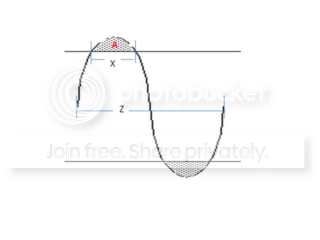# Sine wave segment area computation

Status
Not open for further replies.

#### jeep brainy

##### Newbie level 4
Hi Everyone,
I need to derive a formula for computing an area of a segment within a sine wave.

Given the length of chord (X) and wavelength (Z), how would I get the area of segment A. Many thanks in advance for any help.Cheers,
Jeep

---------- Post added at 06:55 ---------- Previous post was at 06:52 ----------

Last edited:

The problem is about calculating a definite integral ∫sin x - a dx. As a hint: ∫sinx dx = -cos x

Hi FvM,
Please note on those to variables (x and z), I want to compute for the area using them.

cheers,
jeep

The limit angle is: θo = acos(x/(2z))

Then the whole grey area:
A = 4 ∫(cos(x) - cos(θo))dx ; 0,θo

A = 4 (sin(x) - x cos(θo)) |0,θo = 4 ( sin(θo) - θo cos(θo))

A = 4 ( √(1-(x/(2z))²) - (x/(2z)) acos(x/(2z)))

Status
Not open for further replies.